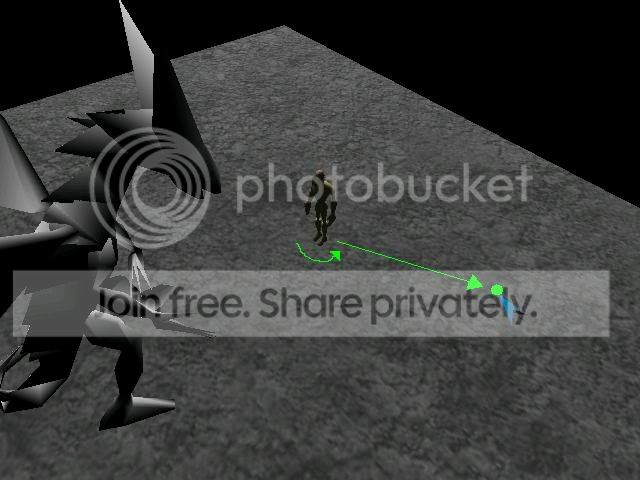# Realy noob question about rotation and translation

Hi!

I note that I have a problem with rotation and translation… :P…

My question is…I want that my character walk to the point clicked by cursor…So, I get the position in the floor, but I cant applay the rotation of my character to the exact point…I put the image to better visualisation…To rotate with keys I use this:

``` if(keyboard.isValidCommand("left")) {          angle += 0.01f;       ```

You can use a method in Vector3f called angleBetween.  You just need two vectors (the direction you are facing and the direction to your target.)  Make sure they are both unit length (normalized) and then call that method to get the angle between them in radians.

Something like this:

``` public void createAngleInRadians(){       this.targetHeading.normalizeLocal();       Vector3f targetPos = targets.getLocalRotation().getRotationColumn(2);       targetPos.normalizeLocal();       this.angle = targetPos.angleBetween(targetHeading);       System.out.println(angle+" : new Angle");    } ```

where:
targetPos <- my character position

I note that "angle" give me a value until 1 phi(180 degree) and not 2phi(360)

Where is the error?

it's 180 because it's the smallest angle between the two and it is expressed positively.  You would use the cross product of your vectors to determine the axis of the rotation which would give you a direction to turn in as well via the sign on the axis.

As an example:

```    public static void main(String[] args) {       Vector3f v1 = new Vector3f(1,0,0).normalizeLocal();       Vector3f v2 = new Vector3f(0,-1,0).normalizeLocal();       Vector3f v3 = new Vector3f(0,1,0).normalizeLocal();              System.err.println("angle 1: "+(FastMath.RAD_TO_DEG * v1.angleBetween(v2)) + " axis: "+v1.cross(v2).normalizeLocal());       System.err.println("angle 2: "+(FastMath.RAD_TO_DEG * v2.angleBetween(v1)) + " axis: "+v2.cross(v1).normalizeLocal());              System.err.println("angle 3: "+(FastMath.RAD_TO_DEG * v1.angleBetween(v3)) + " axis: "+v1.cross(v3).normalizeLocal());       System.err.println("angle 4: "+(FastMath.RAD_TO_DEG * v3.angleBetween(v1)) + " axis: "+v3.cross(v1).normalizeLocal());              System.err.println("angle 5: "+(FastMath.RAD_TO_DEG * v2.angleBetween(v3)) + " axis: "+v2.cross(v3).normalizeLocal());       System.err.println("angle 6: "+(FastMath.RAD_TO_DEG * v3.angleBetween(v2)) + " axis: "+v3.cross(v2).normalizeLocal());    }```

outputs:

```angle 1: 90.0 axis: com.jme.math.Vector3f [X=0.0, Y=0.0, Z=-1.0] angle 2: 90.0 axis: com.jme.math.Vector3f [X=-0.0, Y=0.0, Z=1.0] angle 3: 90.0 axis: com.jme.math.Vector3f [X=0.0, Y=0.0, Z=1.0] angle 4: 90.0 axis: com.jme.math.Vector3f [X=0.0, Y=0.0, Z=-1.0] angle 5: 180.0 axis: com.jme.math.Vector3f [X=-0.0, Y=0.0, Z=0.0] angle 6: 180.0 axis: com.jme.math.Vector3f [X=0.0, Y=0.0, Z=-0.0]```

Notice if you get 0,0,0 (or an angle of 180) the axis is indeterminate, so you'd need to handle that special case.  In such a case, you'd already know what to do in your game though.  Notice also that I normalize everything.  In my case, the values are normalized already, but just to make sure you know they need to be unit length...  :)

Hope that helps.

Hi renanse and everybody!!!

I make this and now is working…more or less…because the update() method make my character crazy… XD…He rotate everytime

Unit length means that the length of your vector is 1.  You can make a vector unit length by calling normalizeLocal.

I notice you are converting your angle to degrees.  How are you using it from there?  If you are using it to make a Quaternion via fromAngleAxis, it needs to be in radians.

What is your targets variable?  Is it the node where your model is under?

If so you would change the last line in what I wrote to targets.setLocalRoatation(q);

When I was debugging my code I would click around my character in a circle to see what would happen.  It was helpful to see at what angles the code failed at.

Good luck.

Hi renanse…

I dont know what I

I did something similar in my game.

What is targetHeading? Is it the position on the floor? Is it a vector from your current position to the target position?

For the parameter to angle between I use a base angle.  Like instead of trying to rotate from where I am to the direction I want to rotate in, I rotate from a starting angle to where I want to be.

This might not be useful, but here is how I would write this function if targetHeading were just the spot you wanted to walk to.

``` private Vector2f baseRot = new Vector2f(1, 0); private Vector3f rotAxis = new Vector3f(0,1,0); public void createAngleInRadians(Vector3f targetHeading){         Vector2f targetPos = new Vector2f();     targetPos.x = targetHeading.x - currentPosition.x;     targetPos.y = targetHeading.y - currentPosition.y;         angulo = targetPos.angleBetween(BaseRot);     if(targetPos.y < 0){       angulo = FastMath.TWO_PI - angulo;       System.out.println(angulo+": Eixo Negativo");     }     else{       System.out.println(angulo+": Eixo Positivo");     }    Quaternion q = new Quaternion();    q.fromAngleAxis(-1*angulo, rotAxis );    setLocalRotation(q); } ```

Hi tandaur!

Yes, the targetHeading is the location in the floor…by your example still dont works in my code…what you do is similar…My character rotate in many positions, sometime in the correct, sometimes no…I guess that I forget something…Hi !!!

using my code, I note the my character get the correct position only in the first time when I click on the floor…

Well put lots of System.out.println's in that method to see whats going on.

You will want to see what targetHeading is at the beginning.

What angulo, targetPos, targets (beginning and end of method), etc.

Then you can see if you are getting the correct angle etc.  It might show you which part of your method is not doing what you think it should do.

Hi !!!

Now is working very well…I change the targetPos to global…:P…

Thx renanse and tandaur for your help!!!